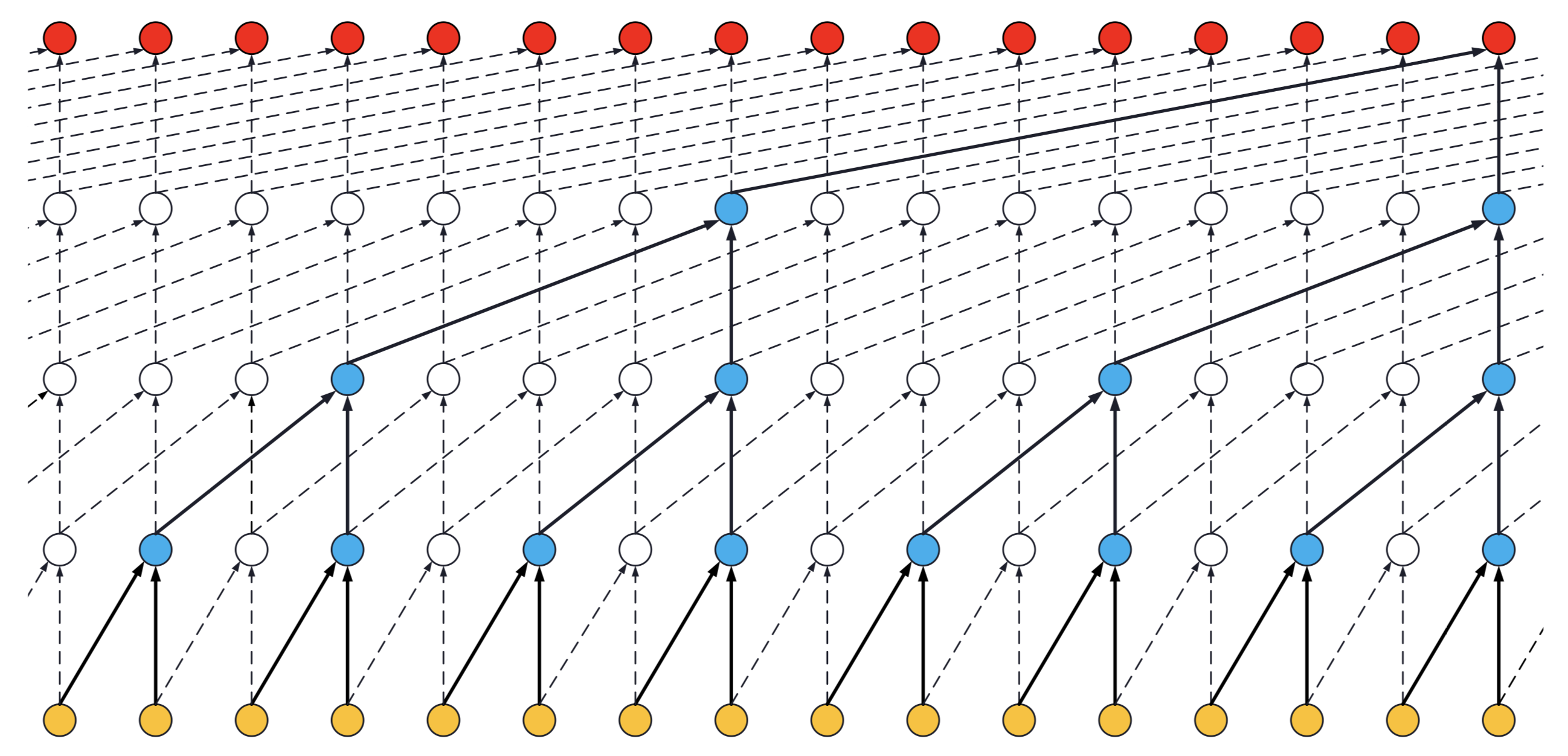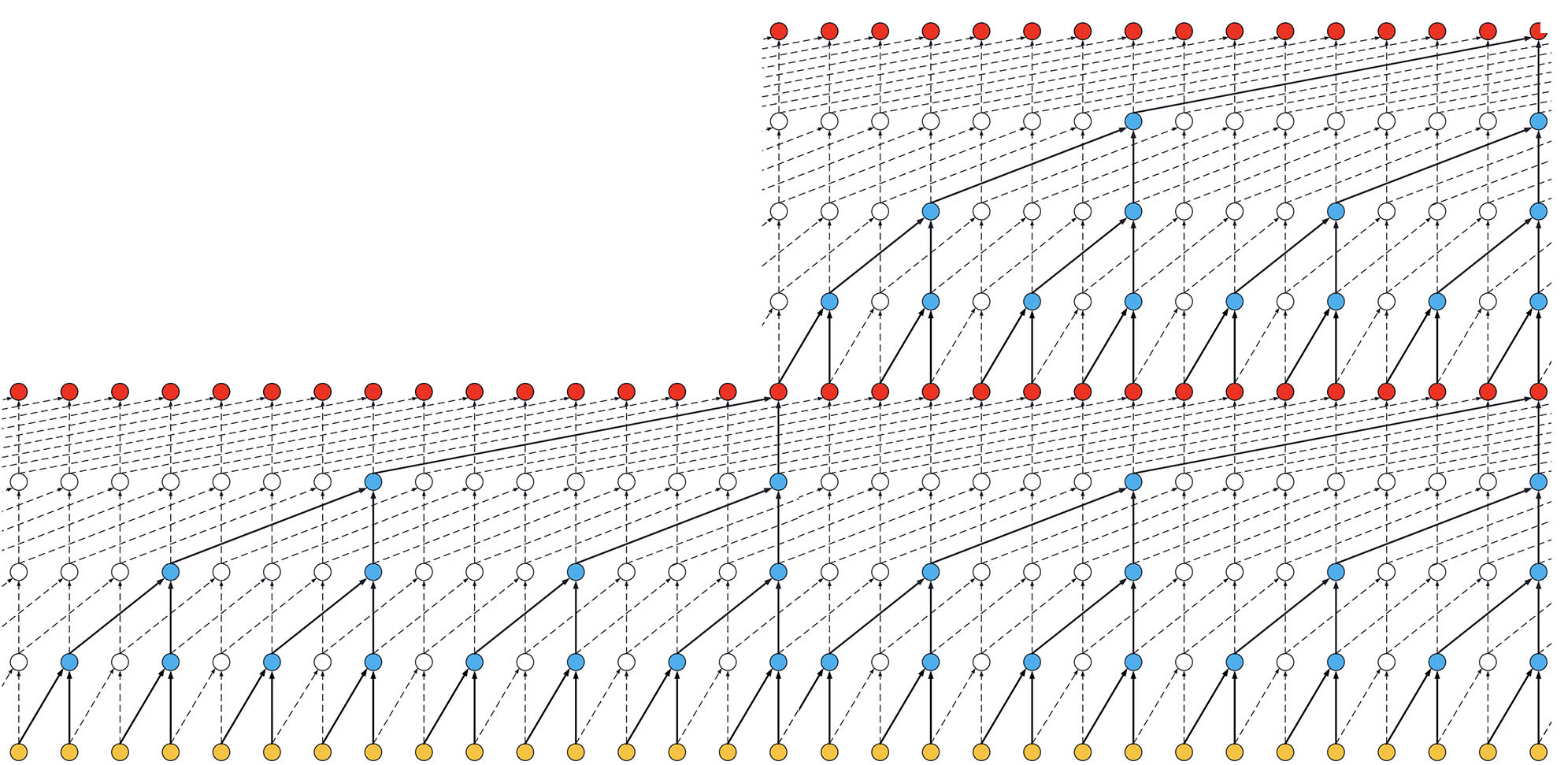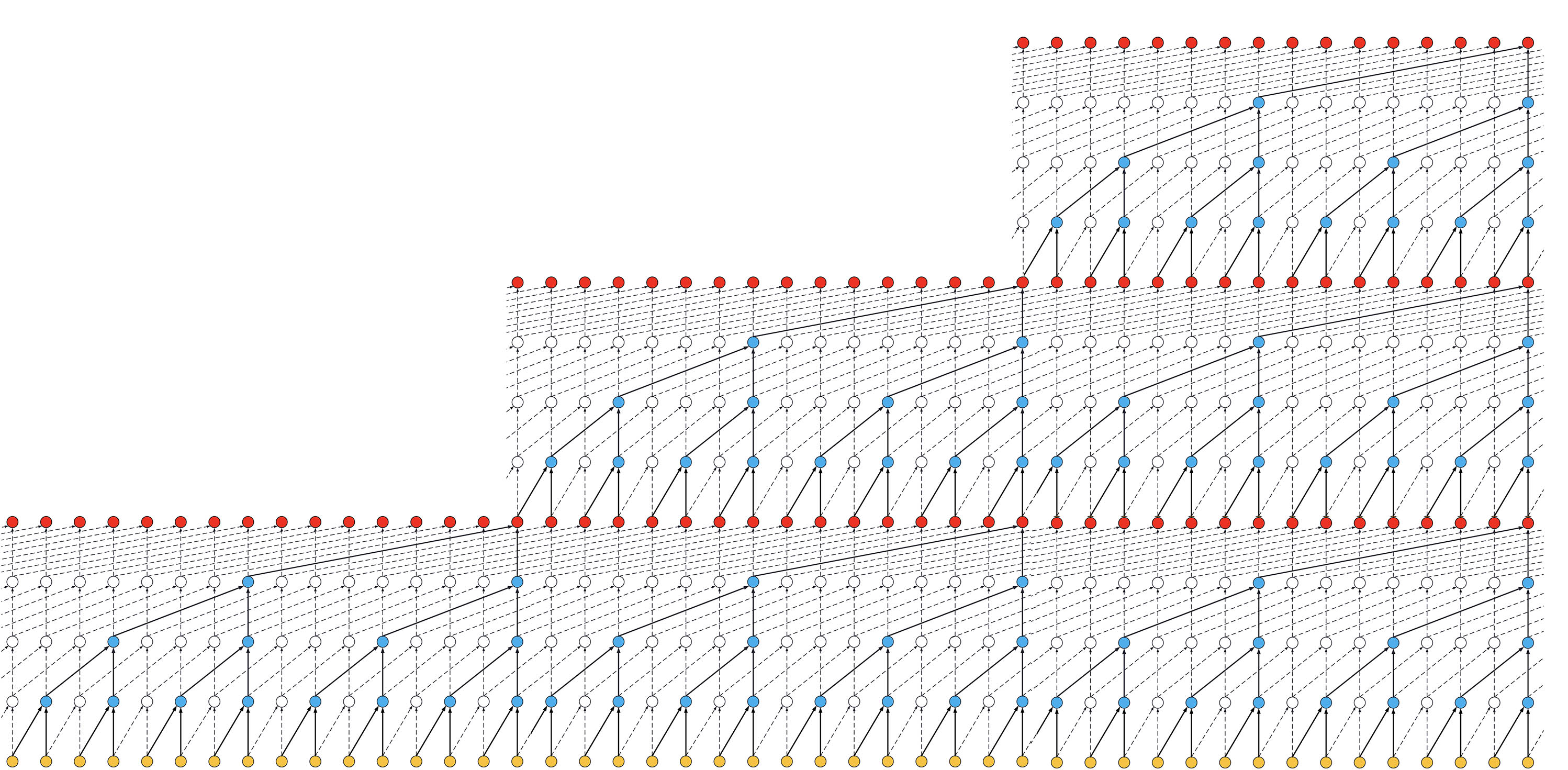# Baichenjia / Tensorflow-TCN

Tensorflow eager implementation of Temporal Convolutional Network (TCN)
PythonFetching latest commit…
Cannot retrieve the latest commit at this time.

## Files

Type Name Latest commit message Commit time
Failed to load latest commit information.adding_problem Mar 18, 2019copy_memory Mar 18, 2019misc Mar 18, 2019mnist_pixel Mar 18, 2019word_ptb Mar 25, 2019LICENSE Mar 25, 2019README.md Mar 18, 2019__init__.pylayer_norm.pytcn.py Mar 18, 2019

# Tensorflow TCN

The explanation and graph in this README.md refers to Keras-TCN.

Temporal Convolutional Network with tensorflow 1.13 (eager execution)

## Why Temporal Convolutional Network?

• TCNs exhibit longer memory than recurrent architectures with the same capacity.
• Constantly performs better than LSTM/GRU architectures on a vast range of tasks (Seq. MNIST, Adding Problem, Copy Memory, Word-level PTB...).
• Parallelism, flexible receptive field size, stable gradients, low memory requirements for training, variable length inputs...Visualization of a stack of dilated causal convolutional layers (Wavenet, 2016)

## API

### Arguments

`tcn = TemporalConvNet(num_channels, kernel_size, dropout)`

• `num_channels`: list. For example, if `num_channels=[30,40,50,60,70,80]`, the temporal convolution model has 6 levels, the `dilation_rate` of each level is `[1, 2, 4, 8, 16, 32]`, and filters of each level are `30,40,50,60,70,80`.
• `kernel_size`: Integer. The size of the kernel to use in each convolutional layer.
• `dropout`: Float between 0 and 1. Fraction of the input units to drop. The dropout layers is activated in training, and deactivated in testing. Using `y = tcn(x, training=True/False)` to control.

### Input shape

3D tensor with shape `(batch_size, timesteps, input_dim)`.

### Output shape

It depends on the task (cf. below for examples):

• Regression (Many to one) e.g. adding problem
• Classification (Many to many) e.g. copy memory task
• Classification (Many to one) e.g. sequential mnist task

### Receptive field

• Receptive field = nb_stacks_of_residuals_blocks * kernel_size * last_dilation.
• If a TCN has only one stack of residual blocks with a kernel size of 2 and dilations [1, 2, 4, 8], its receptive field is 2 * 1 * 8 = 16. The image below illustrates it:ks = 2, dilations = [1, 2, 4, 8], 1 block

• If the TCN has now 2 stacks of residual blocks, wou would get the situation below, that is, an increase in the receptive field to 32:ks = 2, dilations = [1, 2, 4, 8], 2 blocks

• If we increased the number of stacks to 3, the size of the receptive field would increase again, such as below:ks = 2, dilations = [1, 2, 4, 8], 3 blocks

## Run

Each task has a separate folder. Enter each folder one can usually find `utils.py`, `model.py` and `train.py`. The `utils.py` generates data, and `model.py` builds the TCN model. You should run `train.py` to train the model. The hyper-parameters in `train.py` are set by `argparse`. The pre-trained models are saved in `weights/`.

```cd adding_problem/

cd copy_memory/
python train.py # run copy memory task

cd mnist_pixel/
python train.py # run sequential mnist pixel task

cd word_ptb/
python train.py # run PennTreebank word-level language model task```

The training detail of each task is in README.md in each folder.

The task consists of feeding a large array of decimal numbers to the network, along with a boolean array of the same length. The objective is to sum the two decimals where the boolean array contain the two 1s.

The copy memory consists of a very large array:

• At the beginning, there's the vector x of length N. This is the vector to copy.
• At the end, N+1 9s are present. The first 9 is seen as a delimiter.
• In the middle, only 0s are there.

The idea is to copy the content of the vector x to the end of the large array. The task is made sufficiently complex by increasing the number of 0s in the middle.

### Sequential MNIST

The idea here is to consider MNIST images as 1-D sequences and feed them to the network. This task is particularly hard because sequences are `28*28 = 784` elements. In order to classify correctly, the network has to remember all the sequence. Usual LSTM are unable to perform well on this task.

### PennTreebank

In word-level language modeling tasks, each element of the sequence is a word, where the model is expected to predict the next incoming word in the text. We evaluate the temporal convolutional network as a word-level language model on PennTreebank.

## References

You can’t perform that action at this time.